Subscribe to our newsletter to receive the latest news and events from TWI:

# Extreme Value Analysis and Corrosion Mapping Data

## Application of Extreme Value Analysis to Corrosion Mapping Data

Charles SCHNEIDER, TWI, Cambridge, England

Paper presented at 4th European - American Workshop on Reliability of NDE, Berlin, Germany, 24-26 June 2009.

## Abstract

Extreme Value Analysis can be used to extrapolate sample inspection data into uninspected regions of a component. These methods can be used in conjunction with structural reliability software to predict the probability of future failure of the component. This information can help both operators and regulators make risk-based decisions on the future operation of the plant.

This paper illustrates the approach with reference to a case study, based on data collected from a pipeline system in an oil platform using an ultrasonic corrosion mapping technique. Part of the pipeline system was inaccessible for inspection. In this region, therefore, it was necessary to estimate the condition of the pipe based on sample inspections in the accessible area. This was done by partitioning the pipe surface into rectangular blocks and fitting statistical distributions to the minimum wall thicknesses in these blocks. These 'extreme value' distributions were used to theoretically derive the corresponding distributions in the inaccessible part of the system. Structural reliability software was then used to predict the probability of future leakage. Analytic approximations were used both to verify the software and to estimate confidence limits for the predicted probabilities.

The analysis showed that, for the majority of the line, the risk of leakage within its planned lifetime was negligible. However, the work highlighted the need for a better understanding of the rate of corrosion in two particular regions. As a result, a small number of ultrasonic transducers were permanently attached to these two sections of pipe. These transducers have yielded further information on the subsequent rate of corrosion.

The paper will examine the assumptions underlying the analysis. It will discuss how the effect of correlation between neighbouring data points, known as data 'clustering', can be assessed and mitigated against. It will also provide guidance on assessing the goodness-of-fit to the distributions used.

## 1. Introduction

Prior to inspection, the overall condition of the pipeline system was unknown, and corrosion attack was suspected. Inspection of the whole line was not possible, so it was decided that statistical extrapolation would be used to assess those parts of the system that were not inspected. The sample inspection was carried out using ultrasonics.

Figure 1 illustrates the overall pipeline system. Part of the inaccessible pipework is at a higher temperature than the rest of the line, so it may have corroded more than the accessible pipes. We incorporated this 'temperature effect' into our assessment by applying a scaling factor F to the assumed rate of corrosion.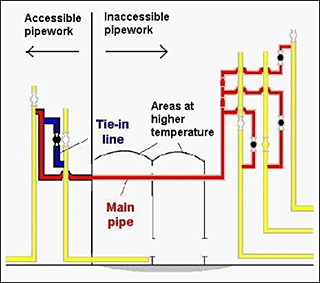Fig.1. General layout of pipeline system

## 2. Objectives

• To analyse results from the inspection of a portion of the line and thereby make an assessment of the whole pipeline system
• To calculate future probability of leakage for the system

## 3. Key assumptions

### 3.1 Applicability of inspection results to uninspected regions

The loss of wall thickness in each uninspected region of the system is assumed to follow the same distribution (after scaling by the 'temperature factor' F where appropriate) as some region that has been inspected.

### 3.2 Ultrasonic measurement errors

The ultrasonic measurement errors are assumed to be negligible. If the systematic (mean) measurement error is zero, any random measurement errors will introduce some conservatism into our estimates of leakage probability, by introducing more scatter in the thickness distributions.

### 3.3 Corrosion rates

• The corrosion rate is assumed not to change with time.
• In extrapolating the leakage predictions to the storage cells, the assumed corrosion rate is increased by a 'temperature factor' F. Our base assumption is that F = 2, but we also investigated the sensitivity of the predictions to the assumed value of F (see below).
• Leakage is assumed not to occur until the minimum thickness reaches zero.

## 4. Extreme value analysis - approach

TWI identified three potential methods for the analysis:

1. Identify a fit to the underlying distribution of the raw data.
2. Partition the pipe surface into rectangular 'blocks', and fit an extreme value distribution to the minimum thicknesses of these blocks.
3. Fit a Generalised Pareto distribution to the K smallest block minima (called exceedances). Reiss and Thomas give detailed guidance on the choice of K (which effectively becomes the sample size).

In practice, we found that different thickness distributions applied to different regions of the line. For example, greater corrosion had occurred at the bottom of low-lying horizontal pipes. Field welds were also treated separately from workshop welds. We identified three distinct regions in the tie-in and nine such regions in the main pipe.

### 4.1 Choice of method

The most suitable method depends on the data. For our data, Method A above was quickly ruled out, because the raw inspection data did not fit any common distribution. If a satisfactory fit to the underlying distribution cannot be identified using Method A, then it is usually possible to instead fit an extreme value distribution directly to the minimum wall thicknesses X measured over rectangular 'blocks' of a certain fixed size. Because Method A was found not to be feasible for our data, Method B was the next to be attempted. We found that Method B was suitable for most of our inspection data, although we did use Method C in just one region of the line. For brevity, we below describe Method B only, but the methodology for Method C is mostly analogous. 

### 4.2 Choice of block size

Most of the statistical theory of extreme values is based on the assumption (sometimes implicit) that individual thickness measurements are statistically independent or, at least, that any correlation between the data is negligible. Stronger correlation between (for example) adjacent data points is described by Reiss and Thomas as 'clustering'.

Methods B and C mitigate this clustering effect, to a certain extent, by reducing the sample to that of the block minima. We thus choose each dimension xi of the block such that pairs of data points separated by a distance xi (in the appropriate direction) are weakly correlated. The strength of the correlation can be gauged from the two-dimensional auto-correlation function (2D ACF). We compute the 2D ACF by successively applying a pair of Fast Fourier transforms (invoking the auto-correlation theorem for two-dimensional transforms).

For our data, the 2D ACFs showed that the correlation is greatest where there is the greatest loss of wall thickness. Figure 2, for instance, illustrates the 2D ACF for the most corroded area of the main pipe. Figure 2a) is a plan view, whereas Figure 2b) is a side elevation, which also provides the key to the shading used in Figure 2a). This degree of correlation would make it difficult to extrapolate the underlying distribution of raw data, even if Method A had revealed an adequate fit. These plots show that pairs of points separated by more than 4 pixels (≈40mm) circumferentially or 16 pixels (≈160mm) circumferentially exhibit a moderate degree of correlation (~60%). Also, the correlation dies away fairly quickly at larger distances.

Plots of the ACF help in choosing an appropriate block size. Figure 2, for instance, justifies our choice of a fixed block area of ~0.007m2 (4x16 pixels) for our analysis of this data set (using Method C). Note that there is always a trade-off between the 'declustering' effect of larger block sizes (together with a better fit to the appropriate distribution), on the one hand, and the resulting increase in sampling errors, on the other hand. For our analyses using Method B, we generally found that a block area of 0.03m2 gave a more satisfactory fit to the extreme value distribution.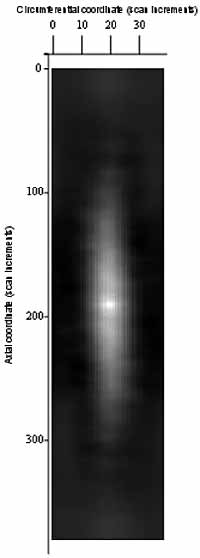a) Plan view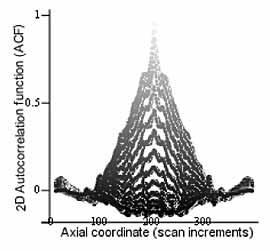b) Side elevation

Fig.2. Two-dimensional auto-correlation function for the most corroded region of the main pipe

### 4.3 Fitting an extreme value distribution to the block minima (Method B)

4.3.1 Probability plot

We can judge how well a Type I extreme value distribution fits the data by examining whether the block minima show a linear trend when plotted on an extreme value probability plot. Figure 3 is an extreme value probability plot of block minima from the most corroded region of the tie-in pipe. The fitted line indicates, for instance, that there is a 5% probability of the minimum thickness over a 0.03m2 patch being less than 11mm. The plotted 95% confidence limits (shown dashed in Figure 3) can also be used as an aid to judgement. In this case, the data show a good fit to an extreme value distribution. Statistical software can then be used to estimate the location and scale parameters (µ and σ) of the distribution, using the maximum likelihood method.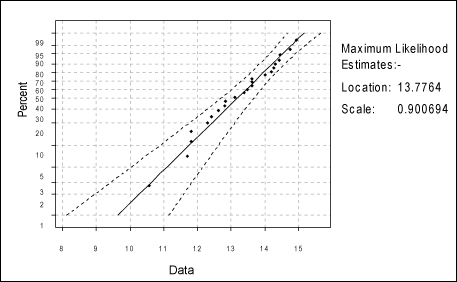Fig.3. Type I extreme value probability plot of minimum thicknesses of 0.03m2 blocks in the most corroded region of the tie-in pipe

4.3.2 Extrapolation over area

The methodology for extrapolating the distribution of minimum thicknesses over area is well established.[4,7] Essentially, the minimum thickness Trem over a larger area A is treated as the minimum of a sample of M independent block minima, where M = A/a and a is the area of each block (Shibata refers to the size factor M as the 'return period'). It then follows that Trem is also distributed according to an extreme value distribution. For a Type I extreme value distribution, for instance, the scale parameter is unchanged (σA=σ), and the location parameter (cf. equation (8) of Shibata is given by:

µA = µ - σloge M     

When extrapolating to a pipe having a reduced initial thickness Thk (relative to that inspected), µA is further reduced by the difference in the (mean) initial thicknesses.

## 5. Initial Assessment (Year 20)

TWI's initial assessment was based solely on inspection data collected after 20 years of operation, based on ultrasonic mapping of the parent pipe and time-of-flight diffraction (TOFD) scans of the welds. No other inspection data was available at this time. Following this inspection, therefore, it was necessary to estimate corrosion rates by assuming:

• Uniform corrosion since start of life, ie initiation of corrosion at Year 0
• A thickness distribution at start of life, derived from the original manufacturing tolerances.

The parameters of (a) the assumed start-of-life distributions, and (b) the extrapolated extreme value distributions at Year 20, were then input to the structural reliability package STRUREL to predict future leakage probabilities.

We checked the leakage probabilities predicted by the STRUREL software using an analytical approximation, by assuming that the initial wall thickness Thk was a fixed value (rather than normally distributed). We assume the corrosion rate is uniform in time, so the maximum loss of wall increases linearly with time, resulting in a simple scaling of the corresponding extreme value distribution. We also obtained approximate confidence limits on the predicted leakage probabilities by applying similar transformations to the confidence limits on the block minima X (such as those plotted in Figure 3).

The results of this initial analysis are summarised in and, for brevity, will not be reproduced here. The analysis showed that, for the majority of the line, the risk of leakage within its planned lifetime was negligible. However, the work highlighted the need for a better understanding of the rate of corrosion in two particular regions:

• The lowest pipe in the tie-in
• Adjacent to the lowest level field welds in the main pipe.

As a result, a small number of ultrasonic transducers were permanently attached to these two sections of pipe. These transducers yielded further information on the rate of corrosion between Year 20 and Year 25.

## 6. Assessment of data from installed system

### 6.1 Analytical approach

A linear statistical model was fitted to the data from the installed system, which took the form:

Y = k + X.B + E    

where Y is wall thickness (the response variable)

k is a regression coefficient ('intercept') estimated from the data by the Maximum Likelihood Method,

X is a vector of factors (including time t, the component, the sensor location and interactions between these factors),

B is a vector of regression coefficients, again estimated from the data, which quantify how strongly wall thickness depends on each factor,

E represents the error in the model, which is assumed to be a random variable, independent of the factors X, and normally distributed with zero mean and constant variance (which is again estimated from the data).

Statistical tests were used to assess those factors that had a significant effect on the wall thickness (at the 5% significance level). These tests indicated that there were three distinct corrosion rates in the tie-in, the main pipe and the field welds of the main pipe. Figure 4 illustrates the sampling distributions of the mean corrosion rate in each of these 'components' of the system.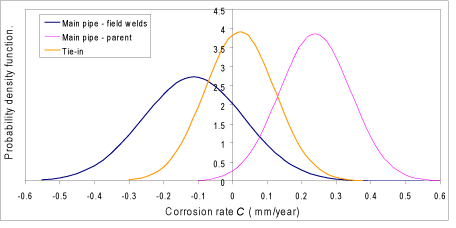Fig.4. General layout of pipeline system

Positive rates in Figure 4 indicate metal loss, while negative rates (e.g. for the bypass field welds) indicate metal gain. Thus Figure 4 shows a high probability of negative corrosion (metal gain) for two of the cases. In fact, in the case of the bypass field welds, analysis of the installed system gives a negative mean corrosion rate and the probability of a negative corrosion rate of 78%. These negative corrosion rates are known a priori to be physically impossible; negative corrosion rates must be due to measurement errors in the installed system. This a priori information was handled in the leakage probability predictions by numerically truncating the distributions at zero. This was carried out using the so-called system reliability component of STRUREL. The resulting leakage probability is, in effect, a conditional probability of the total metal loss equalling the pipe wall thickness at a specified time t, given that the corrosion rate is greater than zero.

Note that Figure 4 illustrates that it is very unlikely that the field welds are corroding more quickly than the base steel, i.e. there is no evidence of preferential weld corrosion.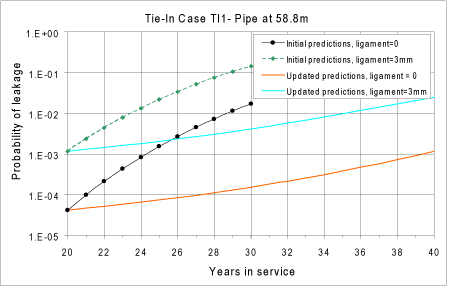Fig.5. Predicted probabilities of leakage in the most corroded region of the tie-in pipe

Within a given component, there were significant variations in wall thickness between different sensors, but no significant variation in corrosion rate (at the 5% level). This supports the view that the extreme value distributions of thicknesses observed at Year 20 (e.g. Figure 3) arose primarily from differences in initiation time rather than differences in corrosion rate.

### 6.2 Leakage probabilities

Figure 5 compares the updated predictions (based on data from the installed system) with the initial predictions made at Year 20 in the most corroded region of the tie-in pipe. The lower pair of curves (those labelled 'ligament=0') correspond to the base assumption that leakage does not occur until the minimum thickness reaches zero. The upper curves (those labelled 'ligament=0') illustrate the sensitivity of the predictions to this assumption; they illustrate the effect of assuming instead that the remaining ligament fails when it reaches a value of 3mm.

In this particular case, the updated leakage probabilities are lower than they were in the initial assessment. This is because the mean corrosion rate measured since Year 20 using the installed system is lower than that assumed previously (based on the assumed distribution of wall thicknesses at start-of-life). However, it is uncertain whether this is due to (i) errors in the previously assumed distribution of wall thicknesses at start-of-life, or (ii) a genuine reduction in the rate of corrosion since Year 20.

## 7. Conclusions

Extreme value statistics provide powerful tools for extrapolating sample inspection data into uninspected regions of a component. These methods can be used in conjunction with structural reliability software to predict the probability of future failure of the component. This information can help both operators and regulators make risk-based decisions on the future operation of the plant.

## 8. Acknowledgements

The author gratefully acknowledges the contributions made by Ms Ruth Sanderson (TWI) and Dr Amin Muhammed (ex-TWI) to this case study. We are also indebted to Dr P J Laycock for originally suggesting Method C (fitting a Generalised Pareto distribution to the exceedances) and for recommending suitable software.

## 9. References

1. Reiss R-D and Thomas M, 'Statistical Analysis of Extreme Values', Birkhäuser Verlag, Basel, 1997.
2. Ripley B D, 'Spatial Statistics', Wiley, New York, 1981. p79.
3. Bracewell R N, 'The Fourier Transform and its Applications', McGraw-Hill, Tokyo, 1978. pp115, 244.
4. Laycock P J, Cottis R A and Scarf P A, 'Extrapolation of Extreme Pit Depth in Space and Time, J. Electrochem. Soc., Vol 137, No 1, pp64-69, January 1990.
5. Joshi N R, 'Statistical Analysis of UT Corrosion Data from Floor Plates of a Crude Oil Aboveground Storage Tank', Materials Evaluation, pp846-849, July 1994.
6. Minitab, 1998: 'Minitab reference manual - Release 12 for Windows'. Minitab Inc (USA), February.
7. Shibata T, 'Application of Extreme Value Statistics to Corrosion', Proc conf 'Extreme value theory and applications', Gaithersburg (1993), Galambos J et al (eds), Vol 2, Journal Research NIST, Washington,1994.
8. Schneider C R A, Muhammed A and Sanderson R M, 'Predicting the remaining lifetime of in-service pipelines based on sample inspection data'. Insight 43 (2) February 2001, pp102-104. Also in Proc. 2000 Annual Br.Conf. on NDT (Buxton).